# Pump Construction (Part1)

In 1689 the physicist Denis Papin invented the centrifugal pump and today this kind of pump is the most used around the world. The centrifugal pump is built on a simple principle: Liquid is led to the impeller hub and by means of the centrifugal force it is flung towards the periphery of the impellers. The construction is fairly inexpensive, robust and simple and its high speed makes it possible to connect the pump directly to an asynchronous motor.

## The centrifugal pump

The centrifugal pump provides a steady liquid flow, and it can easily be throttled without causing any damage to the pump. Now let us have a look at figure 1, which shows the liquid’s flow through the pump. The inlet of the pump leads the liquid to the center of the rotating impeller from where it is flung towards the periphery. This construction gives a high efficiency, and is suitable for handling pure liquids. Pumps, which have to handle impure liquids, such as wastewater pumps, are fitted with an impeller that is constructed especially to avoid that objects get stocked inside the pump.

If a pressure difference occurs in the system while the centrifugal pump is not running, liquid can still pass through it due to its open design. As you can tell from figure 2, the centrifugal pump can be categorized in different groups: Radial flow pumps, mixed flow pumps and axial flow pumps. Radial flow pumps and mixed flow pumps are the most common types used. Therefore, we will only concentrate on these types of pumps.

Different kinds of centrifugal pumps

The different demands on the centrifugal pump’s performance, especially with regards to head, flow, and installation, together with the demands for economical operation, are only a few of the reasons why so many types of pump exist. Figure 3 shows the different pump types with regard to flow and pressure.

## Pump curves

Before we dig any further into the world of pump construction and pump types, we will present the basic characteristics of pump performance curves. The performance of a centrifugal pump is shown by a set of performance curves. The performance curves for a centrifugal pump are shown in figure 4. Head, power consumption, efficiency and NPSH are shown as a function of the flow.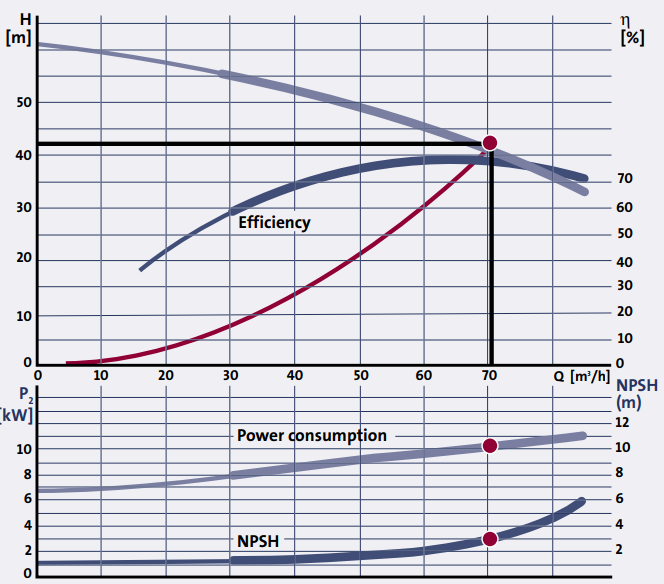Figure 4 – Typical performance curves for a centrifugal pump. Head, power consumption, efficiency and NPSH are shown as a function of the flow

Normally, pump curves in data booklets only cover the pump part. Therefore, the power consumption, the P2 – value, which is listed in the data booklets as well, only covers the power going into the pump – see figure 4. The same goes for the efficiency value, which only covers the pump part (η = ηP ).

In some pump types with integrated motor and possibly integrated frequency converter, e.g. canned motor pumps, the power consumption curve and the η-curve cover both the motor and the pump. In this case it is the P1 -value that has to be taken into account. In general, pump curves are designed according to ISO 9906 Annex A, which specifies the tolerances of the curves.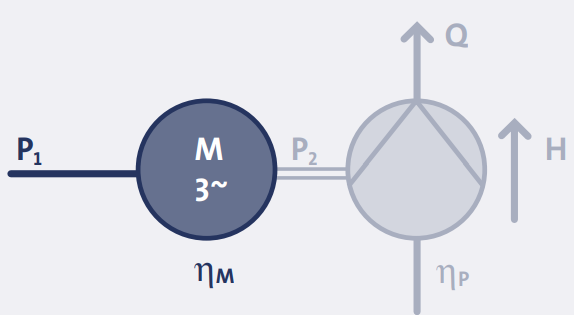Figure 5 – The curves for power consumption and efficiency will normally only cover the pump part of the unit – i.e. P2 and ηP

The QH-curve shows the head, which the pump is able to perform at a given flow. Head is measured in meter liquid column [mLC]; normally the unit meter [m] is applied. The advantage of using the unit [m] as the unit of measurement for a pump’s head is that the QH-curve is not affected by the type of liquid the pump has to handle.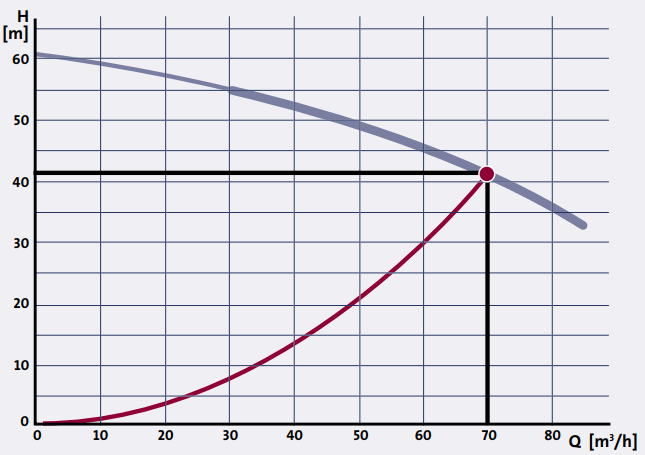Figure 6 – A typical QH-curve for a centrifugal pump; low flow results in high head and high flow results in low head

## Efficiency, the η-curve

The efficiency is the relation between the supplied power and the utilized amount of power. In the world of pumps, the efficiency ηP is the relation between the power, which the pump delivers to the water (PH) and the power input to the shaft (P2 ):

eta _(P)=(P_(H))/(P_(2))=(rho*g*Q*H)/(P_(2))

where:

• ρ is the density of the liquid in kg/m3 ,
• g is the acceleration of gravity in m/s2 ,
• Q is the flow in m3 /s and H is the head in m.

For water at 20oC and with Q measured in m3/h and H in m, the hydraulic power can be calculated as :

P_(H)=2.72*Q*H[W]

As it appears from the efficiency curve, the efficiency depends on the duty point of the pump. Therefore, it is important to select a pump, which fits the flow requirements and ensures that the pump is working in the most efficient flow area.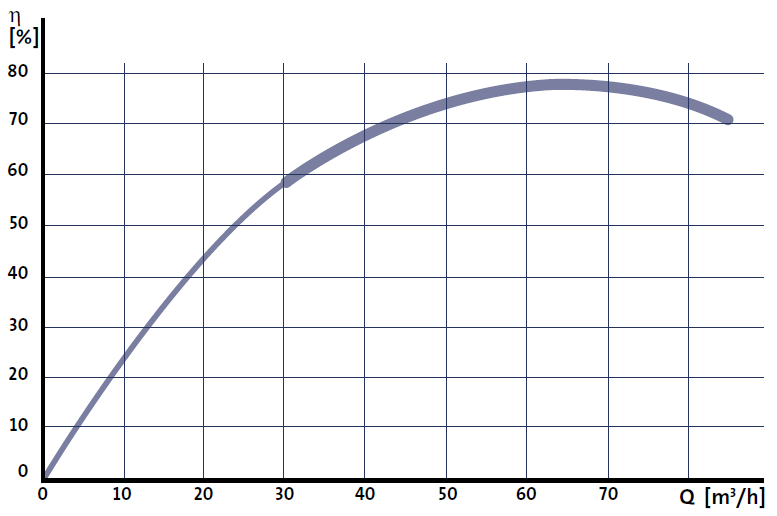Figure 7 – The efficiency curve of a typical centrifugal pump

## Power consumption, the P2-curve

The relation between the power consumption of the pump and the flow is shown in figure 8. The P2-curve of most centrifugal pumps is similar to the one in figure 8 where the P2 value increases when the flow increases.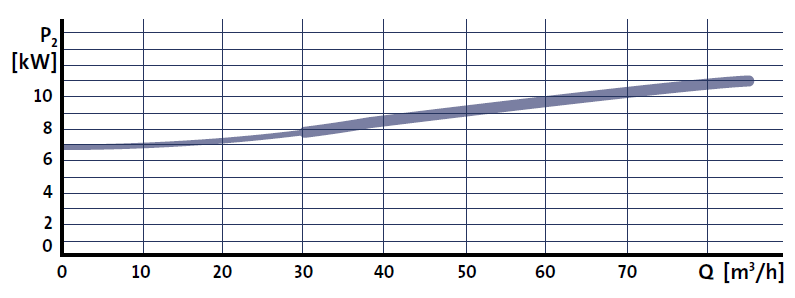Figure 8 – The power consumption curve of a typical centrifugal pump

The NPSH-value of a pump is the minimum absolute pressure that has to be present at the suction side of the pump to avoid cavitation. The NPSH-value is measured in [m] and depends on the flow; when the flow increases, the NPSH-value increases as well; figure 9.